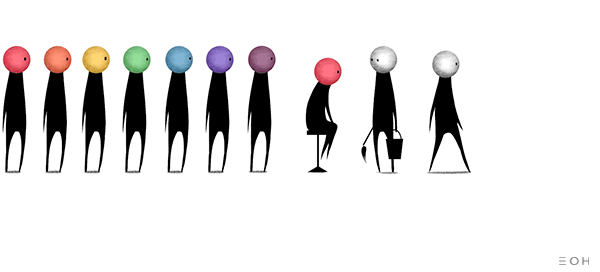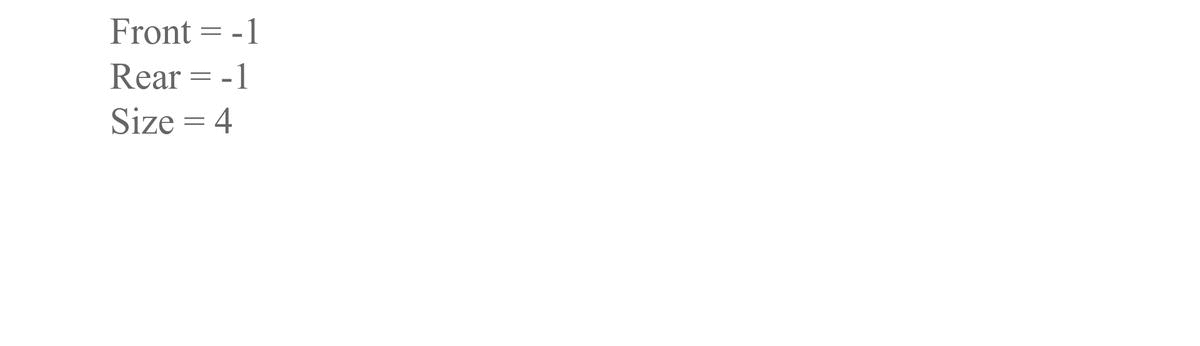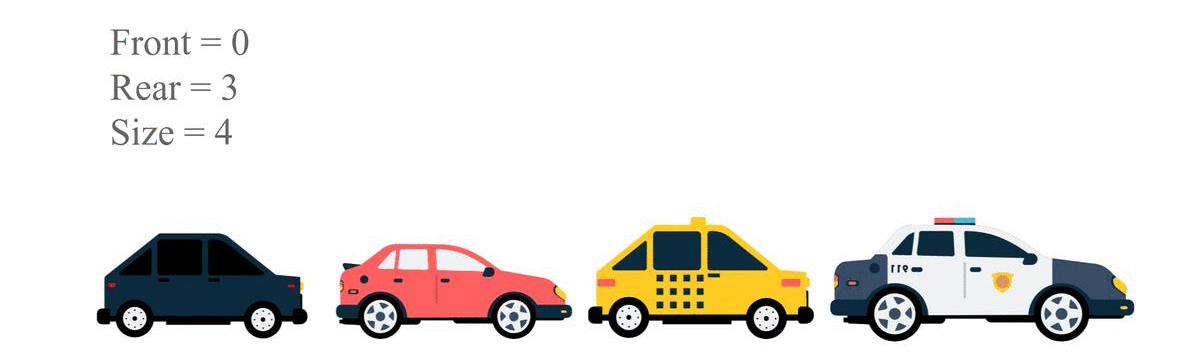# Queues

Written by Richa Kiran on Jul 7th, 2021 Views

A queue is a linear data structure which follows FIFO principle in deletion and insertion operations.

In real sense queue is just a sequence of objects(people, vehicles, etc.) waiting for their turn to come. For instance, it could be a queue of people waiting for their turn to come to buy tickets for a movie show, it could be a queue of vehicles waiting for the traffic signal to turn green, you can imagine more scenarios like that.As you can see, people can join the queue from the back, and the one in the front can get out of it.

So from this we conclude that Insertion is done from the rear end of queue data structure and deletion is done from the front end. The insertion process is called Enqueue and the deletion process is called Dequeue. First of all, lets look at some basic components of a queue.

## Basic structure of a queue

``````typedef struct q{
int front, rear;
int arr[MAX];
int size;
}QUEUE;
``````

The structure contains integers front and rear to keep track of the elements added or deleted from the queue, arr is for storing the elements added, and size is just the user entered size of the queue.

## Enqueue

Enqueue is basically what insert operation is called in a queue. Enqueue or insertion always takes place at the rear end of a queue.

To enqueue an element into the queue, first check if the queue is full or not. If it is full then just print overflow statement. Otherwise increase the rear value of the queue and insert the element at the rear-th index. Note that front and rear are initially set to -1. Steps:

1. Check if queue size is equal to rear-front value of the queue. If it is, it means the queue is full. Print "Overflow!". Otherwise do the following:
1. Check if front value is equal to -1. If so, set it to 0.
2. Increase the value of rear by 1.
3. Add the value at the rear-th index of the array in queue. The C code is follows:
``````void Enqueue(QUEUE *q, int val){
if(q->size-1 == q->rear-q->front){
printf("Queue Overflow!\n");
}
else{
if(q->front==-1){
q->front=0;
}
q->rear++;
q->arr[q->rear] = val;
}
}
``````## Dequeue

Dequeue is the deletion operation of a queue. It always takes place from the front end.

To dequeue and element you have to first check if the queue is empty or not. If so, then print ""Underflow!". Otherwise just increase the value of front by 1. Steps:

1. Check if the front value is greater than rear value or if both are equal to -1. These two conditions indicate that the queue is empty.
2. If so, print "Underflow". Otherwise do the following:
1. increase front by 1.

The C code is as follows:

``````void Dequeue(QUEUE *q){
if((q->front==-1 && q->rear==-1) || (q->front > q->rear)){
printf("Queue Underflow!\n");
}
else{
printf("Dequeuing %d\n", q->arr[q->front]);
q->front++;
}
}
``````## Display

The display function works just like how we print any other array. But first we need to check if the Queue is empty. If it is, print so. Otherwise just print the array using for loop. Steps:

1. Check if the queue is empty.
2. If empty print "empty queue". Else print the array of the queue using for loop. The code -
``````void display(QUEUE *q){
if((q->front==-1 && q->rear==-1) || (q->front > q->rear)){
printf("Empty queue!\n");
}
else{
printf("QUEUE: ");
for(int i=q->front; i<=q->rear; i++){
printf("%d ", q->arr[i]);
}printf("\n");
}
}
``````

## Full Code:

``````#include <stdio.h>
#include <stdlib.h>
#define MAX 10

typedef struct q{
int front, rear;
int arr[MAX];
int size;
}QUEUE;

void Enqueue(QUEUE *q, int val){
if(q->size-1 == q->rear-q->front){
printf("Queue Overflow!\n");
}
else{
if(q->front==-1){
q->front=0;
}
q->rear++;
q->arr[q->rear] = val;
}
}

void Dequeue(QUEUE *q){
if((q->front==-1 && q->rear==-1) || (q->front > q->rear)){
printf("Queue Underflow!\n");
}
else{
printf("Dequeuing %d\n", q->arr[q->front]);
q->front++;
}
}

void display(QUEUE *q){
if((q->front==-1 && q->rear==-1) || (q->front > q->rear)){
printf("Empty queue!\n");
}
else{
printf("QUEUE: ");
for(int i=q->front; i<=q->rear; i++){
printf("%d ", q->arr[i]);
}printf("\n");
}
}

int main(){
int n;
printf("Enter the size of queue: ");
scanf("%d", &n);
QUEUE *q = (QUEUE*)malloc(n*sizeof(QUEUE));
q->front = -1;
q->rear = -1;
q->size = n;
int ch;
do{
printf("\tEnter\n\t1.Enqueue\n\t2.Dequeue\n\t3.Display\n\t4.Exit\n");
printf("Enter choice: ");
scanf("%d", &ch);
switch(ch){
case 1:
{
//Enqueue
printf("Enter the value to be enqeued: ");
int val;
scanf("%d", &val);
Enqueue(q,val);
break;
}
case 2:
{
//Dequeue
Dequeue(q);
break;
}
case 3:
{
//Display
display(q);
break;
}
case 4:
{
//exit
printf("Exiting...\n");
break;
}
default:
{
printf("Enter valid choice!\n");
break;
}
}
}while(ch!=4);

}
``````